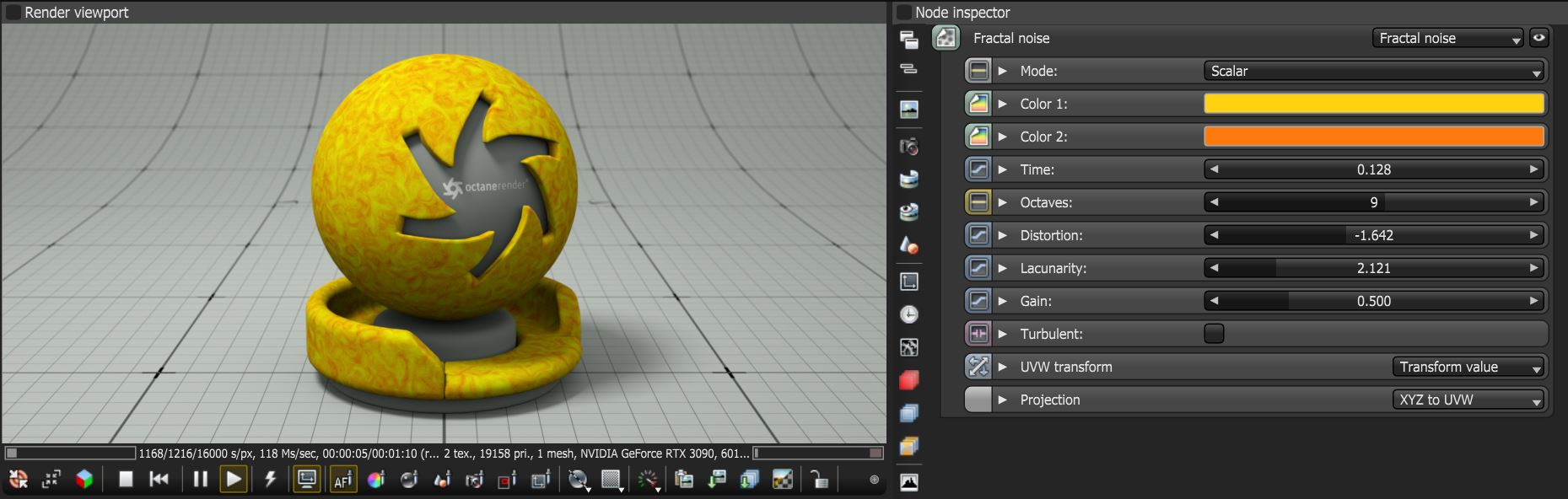# Fractal Noise

The Fractal Noise texture node generates a fractal-based pattern (figure 1). The parameters consist of UVW Transform and Projection inputs which can be used to edit the pattern's position and scale.Figure 1: The Fractal node and it's associated parameters

### Fractal Noise Parameters

Mode - Specifies whether scalar or vector mode is used to calculate the fractal noise.

Colors 1 & 2 - Specifies the two colors used to generate the fractal noise.

Time - Shifts the fractal pattern over time.

Octaves - Specifies the multiple frequencies in which the fractal pattern is repeated.

Distortion - Determines the scale of random displacement applied to the fractal pattern.

Lacunarity - Controls the size of the space in the fractal texture pattern.

Gain - An amplitude multiplier per iteration where higher values will result in a flatter pattern.

Turbulent - Adds an additional layer of noise to the fractal pattern.

UVW Transform - Positions, scales, and rotates the surface texture.

Projection - Sets how the texture projects onto the surface.